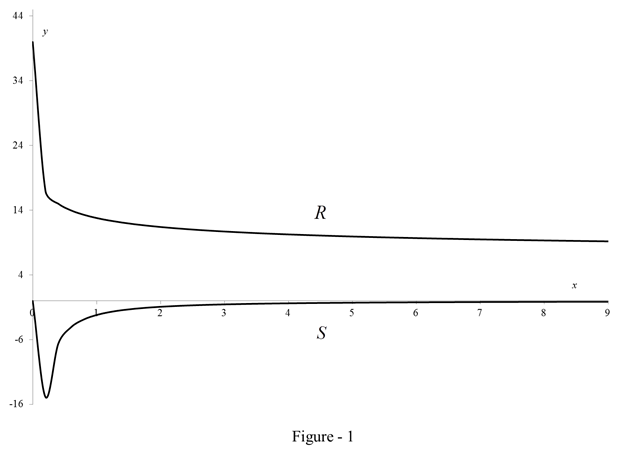# The sensitivity of the body.### Single Variable Calculus: Concepts...

4th Edition
James Stewart
Publisher: Cengage Learning
ISBN: 9781337687805### Single Variable Calculus: Concepts...

4th Edition
James Stewart
Publisher: Cengage Learning
ISBN: 9781337687805

#### Solutions

Chapter 3.8, Problem 32E

(a)

To determine

## To find: The sensitivity of the body.

Expert Solution

Sensitivity of the body is S=54.4x0.6(1+16x0.8+8x0.4).

The graph is plotted between x and y coordinates for both R and S is shown in figure (1).

### Explanation of Solution

Given:

The experimental formula is shown below.

R=40+24x0.41+4x0.4 (1)

Calculation:

Calculate the sensitivity S.

S=dRdx

Sensitivity is defined to be the rate of change of the reaction with respect to x.

Substitute 40+24x0.41+4x0.4 for R in the above equation.

S=ddx(40+24x0.41+4x0.4)

Apply the quotient rule below.

(uv)'=u'vv'uv2

Substitute (40+24x0.4) for u and (1+4x0.4) for v in the above equation.

S=(40+24x0.4)'(1+4x0.4)(1+4x0.4)'(40+24x0.4)(1+4x0.4)2=9.6x0.6(1+4x0.4)1.6x0.6(40+24x0.4)(1+4x0.4)2=1.6x0.6(6(1+4x0.4)(40+24x0.4))(1+4x0.4)2=1.6x0.6(6+24x0.44024x0.4)(1+(4x0.4)2+2(1)(4x0.4))

S=54.4x0.6(1+16x0.8+8x0.4) (2)

Thus, the sensitivity of the body is 54.4x0.6(1+16x0.8+8x0.4).

(b)

To determine

Expert Solution

### Explanation of Solution

Illustration:

Sketch the curve.

Calculate the value of R using the equation (1).

R=40+24x0.41+4x0.4

Substitute 0 for x in the equation (1).

R=40+24(0)0.41+4(0)0.4=40

Repeat the calculation of the value R for values of x from 0.2 till 10.

Tabulate the value of x and R as shown in table (1).

 x R=40+24x0.41+4x0.4 0.00 40.00 0.20 16.96 0.40 15.01 0.60 13.98 0.80 13.30 1.00 12.80 1.20 12.41 1.40 12.10 1.60 11.83 1.80 11.61 2.00 11.42 2.20 11.24 2.40 11.09 2.60 10.95 2.80 10.83 3.00 10.72 3.20 10.61 3.40 10.52 3.60 10.43 3.80 10.35 4.00 10.27 4.20 10.20 4.40 10.13 4.60 10.06 4.80 10.00 5.00 9.95 5.20 9.89 5.40 9.84 5.60 9.79 5.80 9.74 6.00 9.70 6.20 9.66 6.40 9.62 6.60 9.58 6.80 9.54 7.00 9.50 7.20 9.47 7.40 9.43 7.60 9.40 7.80 9.37 8.00 9.34 8.20 9.31 8.40 9.28 8.60 9.25 8.80 9.22 9.00 9.20 9.20 9.17 9.40 9.15 9.60 9.12 9.80 9.10 10.00 9.08

Calculate the value of S using the equation (2).

S=54.4x0.6(1+16x0.8+8x0.4)

Substitute 0 for x in the equation (1).

S=54.4(0)0.6(1+16(0)0.8+8(0)0.4)=0

Repeat the calculation of the value S for values of x from 0.2 till 10.

Tabulate the value of x  and S as shown in table (2).

 x S=−54.4x−0.6(1+16x0.8+8x0.4) 0.00 0 0.20 -14.856 0.40 -6.6235 0.60 -4.0713 0.80 -2.8659 1.00 -2.176 1.20 -1.7342 1.40 -1.4297 1.60 -1.2083 1.80 -1.041 2.00 -0.9106 2.20 -0.8064 2.40 -0.7215 2.60 -0.6512 2.80 -0.592 3.00 -0.5417 3.20 -0.4984 3.40 -0.4609 3.60 -0.428 3.80 -0.399 4.00 -0.3733 4.20 -0.3503 4.40 -0.3298 4.60 -0.3112 4.80 -0.2944 5.00 -0.2791 5.20 -0.2651 5.40 -0.2524 5.60 -0.2406 5.80 -0.2298 6.00 -0.2198 6.20 -0.2105 6.40 -0.2019 6.60 -0.1939 6.80 -0.1864 7.00 -0.1795 7.20 -0.1729 7.40 -0.1668 7.60 -0.161 7.80 -0.1556 8.00 -0.1505 8.20 -0.1456 8.40 -0.1411 8.60 -0.1367 8.80 -0.1326 9.00 -0.1288 9.20 -0.1251 9.40 -0.1215 9.60 -0.1182 9.80 -0.115 10.00 -0.112

Graph:

Sketch the curve using table (1) and table (2) as shown in figure (1).Refer the figure (1).

For all the small values of x, we have R reaching a value near 40, which is quite high. So a small stimulus produces a large reaction which is something to except.